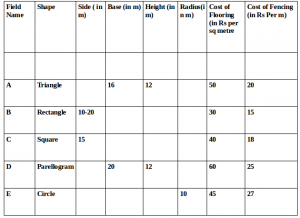## Data Interpretation For SBI PO : Set – 36

D.1-5) Study the given table carefully to answer the following questions.1) What is the cost of flooring of A?

a) Rs.4000

b) Rs.4600

c) Rs.4800

d) Rs.5000

e) Rs.4400

Click Here to View Answer

c)

A is a triangle

So area of A = 1/2*16*12= 96sqm

So, cost of flooring of A = 96*50 = Rs.4800

2) What is the difference between the cost of fencing of C and that of B?

a) Rs.180

b) Rs.120

c) Rs.240

d) Rs.360

e) Rs.480

Click Here to View Answer
a)

Perimeter of B = 2(10+20) = 60m

So,cost of fencing of B = 60*15= 900

Perimeter of C = 4*15 = 60m

So cost of fencing of C = 60 *18 = Rs.1080

So,required difference = 1080 – 900 = Rs.180

3) The cost of fencing of field E is approximately what percent of the cost of flooring of field C?

a) 10.5%

b) 19.46%

c) 18.71%

d) 15.36%

e) 13.82%

Click Here to View Answer
d)

Perimeter of E = 2πr = 2 × 22 / 7 × 10 = 440 / 7 m

Cost of fencing of E =  440 / 7 × 22 = Rs.1382.85

Area of C = (15)2 = 225m2

So, cost of flooring of C = 225 × 40 = Rs.9000

So, required % = 1382.85 × 100 / 9000

= 15.36%

4) The cost of fencing of field C is what percent of the cost of fencing of field D?

a) 87.54%

b) 67.5%

c) 72.13%

d) 54.36%

e) 46.5%

Click Here to View Answer

b)

Fencing cost of C = Rs.1080

Fencing cost of D = Rs.1600

Required % =  1080 / 1600 × 100 = 67.5%

5) What is the ratio of the cost of flooring to that of fencing of field D?

a) 4 : 1

b) 6 : 1

c) 8 : 1

d) 9 : 1

e) 5 : 1

Click Here to View Answer

d)

Area of D = Base × Height = 20 × 12 = 240m2

So, cost of flooring of D= 240 × 60 = Rs.14400

Perimeter of D = 2 (20 + 12) = 64 m

So, cost of fencing of D = 64 × 25 = Rs.1600

So, required ratio = 14400 : 1600 = 9 : 1

D.6-10) The table below shows the estimated cost (in Rs. Lakh) of a project of laying a railway line between two places.6) The total expenditure is required to be kept within Rs. 700 lakh by cutting the expenditure on administration equally in all the years. What will be the percentage cut for 1989?

a) 22.6

b) 32.6

c) 42.5

d) 52.6

e) None of these

Click Here to View Answer

c)

total expenditure = 52.1+267.5+196.4+209.5 = 725.5 lakhs.

the question states that the expenditure has to be limited to/kept within 700 lakhs.

This means that the total expenditure has reduced by 25.5 lakhs.

Thus, each year, the expenditure has to reduce by = (25.5/4) = 6.375 lakhs.

Therefore, percentage reduce for administration in 1989 would be = (6.375/15) × 100 = 42.5%.

7) If the length of line to be laid each year is in proportion to the estimated cost for material and labour, what fraction of the total length is proposed to be completed by the third year?

a) 0.9

b) 0.7

c) 0.6

d) 0.3

e) None of these

Click Here to View Answer
b)

Cost for 1988 = 2.1

Cost for 1989 = 95+70+15+25+25 = 230

Cost for 1990 = 80+45+12+18+20 = 175

Cost for 1991 = 75+60+16+21+18 = 190

Thus, proportion of these expenditures till 1990 = (2.1 + 230 + 175) / (2.1 + 230 + 175 + 190) = 0.6817. Remember, this also will be be the fraction of the total length of the line.

8) If the cost of materials rises by 5% each year from 1990 onwards, by how much will the estimated cost rise?

a) Rs. 11.4 lakh

b) Rs. 16.4 lakh

c) Rs.21.4 lak

d) Rs.26.4 lakh

e) None of these

Click Here to View Answer

b)

The estimated cost in 1990 = 80 + 45 + 12 + 18 = 155

The estimated cost in 1991 = 75 + 60 + 16 + 21 = 172

Cost of material rises by 5% in each year. This means that the individual cost for the two years, 1990 and 1991

increases by 5% each. This, the increase in cost= 0.05 × (155 + 172) = Rs.16.35 lakhs.

9) What is the approximate ratio of the total cost of materials for all the years bear to the total labour cost?

a) 4 : 1

b) 8 : 1

c) 12:1

d) 16 :1

e) None of these

Click Here to View Answer
b)

The total material cost is (using values from the given table) = (95+80+75+70+45+60+15+12+16+25+18+21) = 532

The total labour cost is (using values from the given table) = (2.1+25+20+18) = 65.1

Now, calculating the ration: = 532 : 65.1

This is approximately = 8 : 1

10) It is found at the end of 1990, that the entire amount estimated for the project has been spent. If for 1991, the actual amount spent was equal to that which was estimated, by what percent (approximately) has the actual expenditure exceeded the estimated expenditure?

a) 39

b) 29

c) 1

d) 9

e) None of these

Click Here to View Answer
b)

The language of this question is tricky and you need to careful.

The total estimated cost of the project is (till the year 1991)= Rs.725.5 lakhs

Now this amount has already been spent.

The estimated Expenditure for 1991 = 209.5 lakhs.

This is the additional amount that will have to be spent on the project.

Thus, the increase in expenditure will be 209.5 on 725.5 = 28.87%.